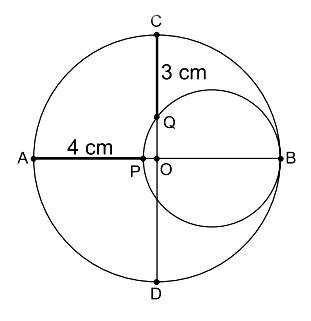# Ascending Crescent

Geometry Level 2Diagram above shows two circles are tangent internally to each other. The straight lines $AB$ and $CD$ are perpedicular and the point $O$ is the center of the larger circle. The length of straight lines $AP$ and $CQ$ are $4\text{ cm}$ and $3\text{ cm}$ respectively. What is the radius of the smaller circle?

×